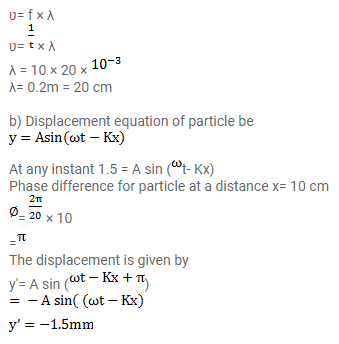# A wave travelling on a string at a speed of 10 m/s causes

Question:

A wave travelling on a string at a speed of $10 \mathrm{~m} / \mathrm{s}$ causes each particle of the string to oscillate with a time period of 20 ms.

a. What is the wavelength of the wave

b. If the displacement of a particle is $1.5 \mathrm{~mm}$ at a certain instant, what will be the displacement of a particle $10 \mathrm{~cm}$ away from it at the same instant?

Solution: